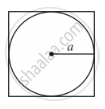Share

# The Perimeter (In Cm) of a Square Circumscribing a Circle of Radius a Cm, is - Mathematics

Course
ConceptCircles Examples and Solutions

#### Question

The perimeter (in cm) of a square circumscribing a circle of radius a cm, is

• A. a

• B. a

• C. a

• D. 16 a

#### SolutionThe radius of the circle is given as a cm.

∴ Diameter of the circle = 2 × a cm = 2a cm

Side of the circumscribing square = Diameter of the circle = 2a cm

Perimeter of the circumscribing square = 4 × 2a cm = 8a cm

Is there an error in this question or solution?

#### Video TutorialsVIEW ALL 

Solution The Perimeter (In Cm) of a Square Circumscribing a Circle of Radius a Cm, is Concept: Circles Examples and Solutions.
S